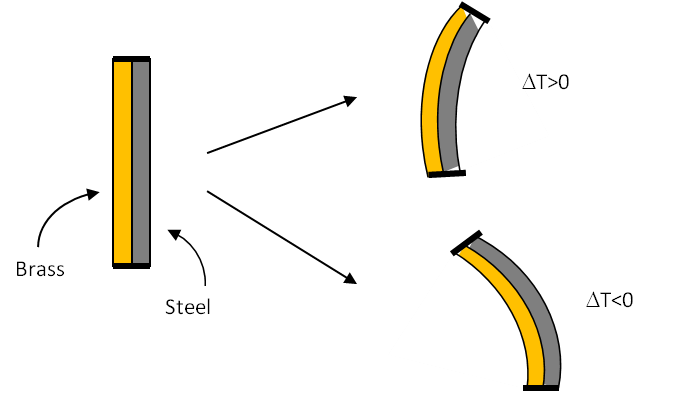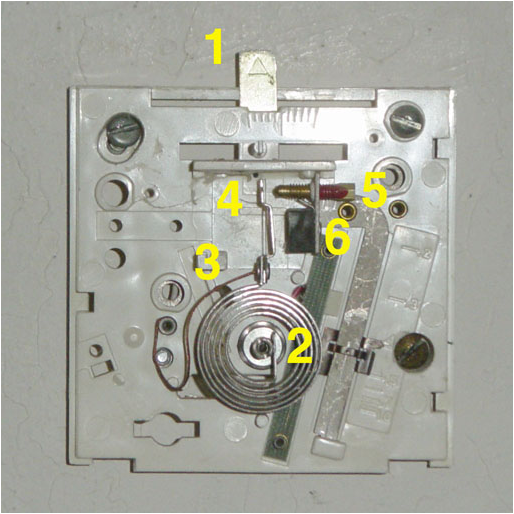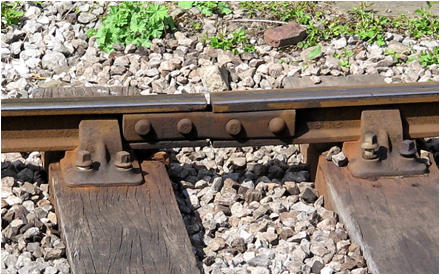## Section20.1Thermal Length Expansion

Most things expand when heated and contract when cooled. When a solid bar is heated its length expands and contracts if cooled if either end is free. In almost all materials, the fractional change in length $\Delta L / L\text{,}$ i.e., the strain, is proportional to the change in temperature $\Delta T \text{.}$

\begin{equation} \dfrac{\Delta L}{L} = \alpha \Delta T,\tag{20.1.1} \end{equation}

where the proportionality constant $\alpha$ is called the coefficient of thermal expansion. Table 20.1.1 has values of the coefficient for common materials. You can see that $\alpha$ is very small for most solids, being $10^{-5}-10^{-6}\text{ per }^\circ\text{C}\text{.}$

### Subsection20.1.1Thermal Expansion Applications

#### Subsubsection20.1.1.1Bimetal Strip

An important application of thermal expansion is a bimetallic strip, which is used to measure temperature. In a bimetallic strip, two strips made up of different metals such as brass and steel are welded at the ends as shown in Figure 20.1.2. When the bimetallic strip is heated the two metals expand to different extent, which causes the bimetal strip to bend since the ends are fixed. The direction of bending can be calibrated to give readings of temperature.Figure 20.1.2. A bimetal strip - the two metals expand with different coefficients, causing the strip to bend one way or the other depending on the temperature.

A common approach for enhancing the effect so that a more sensitive equipment for measuring temperature can be constructed is to wind a bimetallic strip as a helix on a support. The winding lets one use a very long bimetallic wire so that the twists along the wire can be magnified. With temperature the helix winds and unwinds which is displayed on an analog dial or converted to a digital output (Figure 20.1.3).Figure 20.1.3. A bimetal coil in a thermostat.

#### Subsubsection20.1.1.2Preventing Rail Tracks from Stress

Rail tracks are joined together with a gap left for allowing the expansion in hot weather and/or heating of the rails from the friction.Figure 20.1.4. Rail tracks have gaps placed on purpose to prevent the bending of tracks as a result of heat and expansion.

How much gap should be left between two $20\text{-m}$ tracks made of steel if it is laid at $22^{\circ}\text{C}$ and is to operate up to a temperature of $47^{\circ}\text{C}\text{?}$

Each track will expand half of the gap on each side. Hence the total expansion will the equal to the gap.

\begin{align*} \text{Gap } \amp = \Delta L = \alpha L \Delta T \\ \amp = 11.7\times 10^{-6} \times 20 \times 25 = 5.9\times 10^{-3} \text{m}. \end{align*}

An aluminum and a copper bar each of length $1.0\text{ m}$ at $27^{\circ}\text{C}$ are put in boiling water at $99^{\circ}\text{C}\text{.}$ What is the difference in their lengths at $99^{\circ}\text{C}\text{?}$

Data: $\alpha_{\text{aluminum}} = 23.1\times 10^{-6}\ \text{per }^{\circ}\text{C}$ and $\alpha_{\text{copper}} = 16.5\times 10^{-6}\ \text{per }^{\circ}\text{C}\text{.}$

Hint

Use $\Delta L = \alpha L_0 \Delta T\text{.}$

$0.47\text{ mm}.$

Solution

Using $\Delta L = \alpha L_0\Delta T,$ we find the change in the lengths of the two bars when their temperatures are raised to $99^{\circ}\text{C}\text{.}$

\begin{align*} \amp \Delta L_{\text{aluminum}} = \frac{23.1\times 10^{-6}}{\ ^{\circ}\text{C}}\times 1\ \text{m} \times (99-27)^{\circ}\text{C} = 1.66\ \text{mm}.\\ \amp \Delta L_{\text{copper}} = \frac{16.5\times 10^{-6}}{\ ^{\circ}\text{C}}\times 1\ \text{m} \times (99-27)^{\circ}\text{C} = 1.19\ \text{mm}. \end{align*}

Therefore, the difference in the lengths of the the two bars at $99^{\circ}\text{C}$ will be $1.66\text{ mm} - 1.19\text{ mm} = 0.47\text{ mm}\text{.}$

A train track is made of long steel bars each of length $20\text{ m}$ at $20^{\circ}\text{C}\text{.}$ The track is to operate between the temperatures of $-5^{\circ}\text{C}$ and $40^{\circ}\text{C}\text{.}$ What gap should be placed between the tracks at $20^{\circ}\text{C}$ so that the maximum separation between them does not exceed $5\text{ m}$ and the minimum gap be zero in the given temperature range.

Hint

Use $\Delta L = \alpha L_0 \Delta T\text{.}$

Between $0.5\text{ cm}$ and $4.4\text{ cm}\text{.}$

Solution

Let $L_1$ and $L_2$ denote the smallest and largest lengths of the bar in the given temperature range. These lengths of the bar at the two extreme temperatures will be

\begin{align*} L_1 \amp = 20\ \text{m} - \frac{11.7\times 10^{-6}}{\ ^{\circ}\text{C}}\times 20\ \text{m} \times (20-(-5))^{\circ}\text{C}\\ \amp = 19.994\ \text{m}.\\ L_2 \amp = 20\ \text{m} + \frac{11.7\times 10^{-6}}{\ ^{\circ}\text{C}}\times 20\ \text{m} \times (40-20)^{\circ}\text{C}\\ \amp = 20.005\ \text{m}. \end{align*}

Let $w$ be the gap at $20^{\circ}\text{C}\text{.}$ The gap in winter will increase by $0.6 \text{ cm}$ becoming $w + 0.6 \text{ cm}\text{,}$ which is required by the problem not to exceed $5 \text{cm}\text{.}$ Therefore.

\begin{equation*} w \lt 5\ \text{cm} - 0.6\ \text{cm} = 4.4\ \text{cm}. \end{equation*}

In the summer the gap will decrease by $0.5\text{ cm}$ becoming $w - 0.5\text{ cm}\text{,}$ which must not be less than zero since then the rails will push against each other.

\begin{equation*} w \gt 0.5\ \text{cm}. \end{equation*}

Therefore, any gap between $0.5\text{ cm}$ and $4.4\text{ cm}$ will satisfy the requirements.